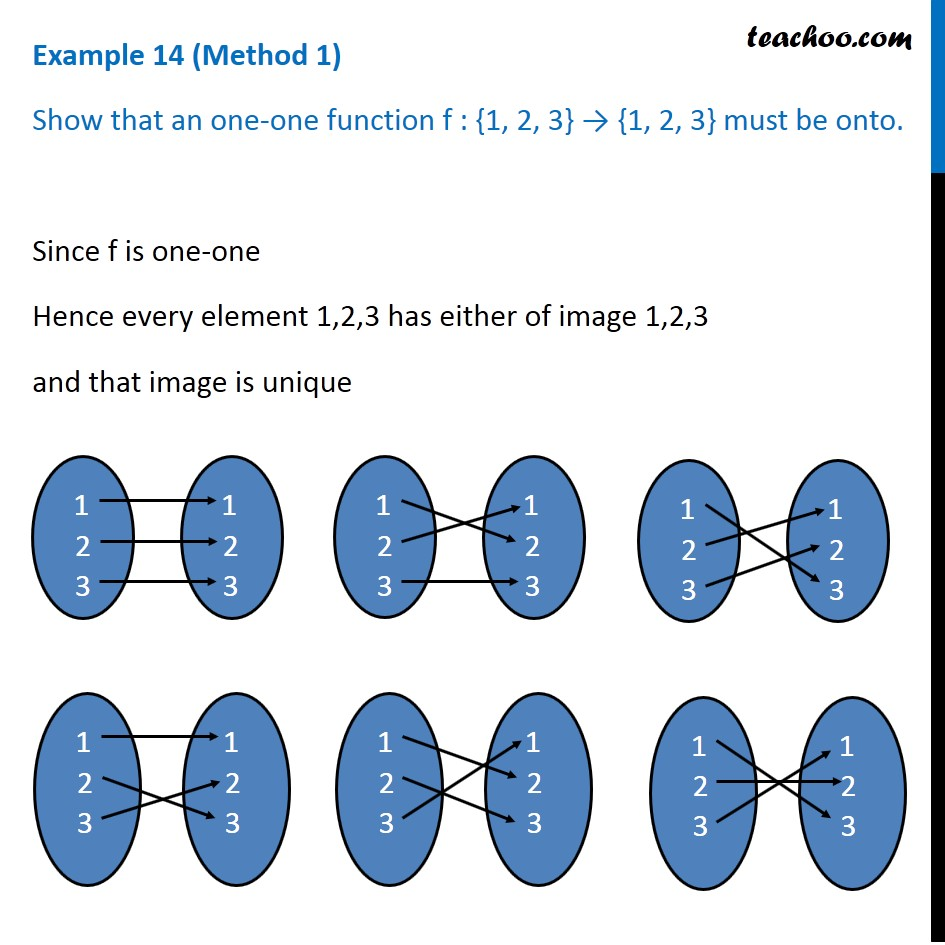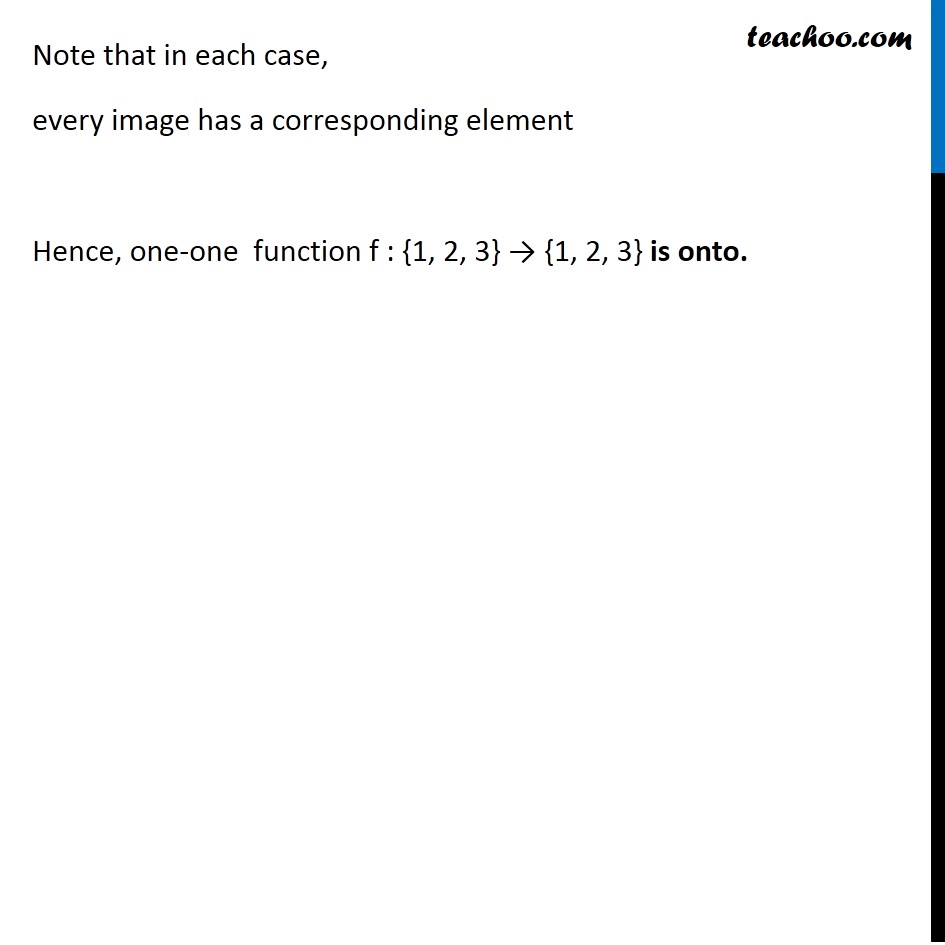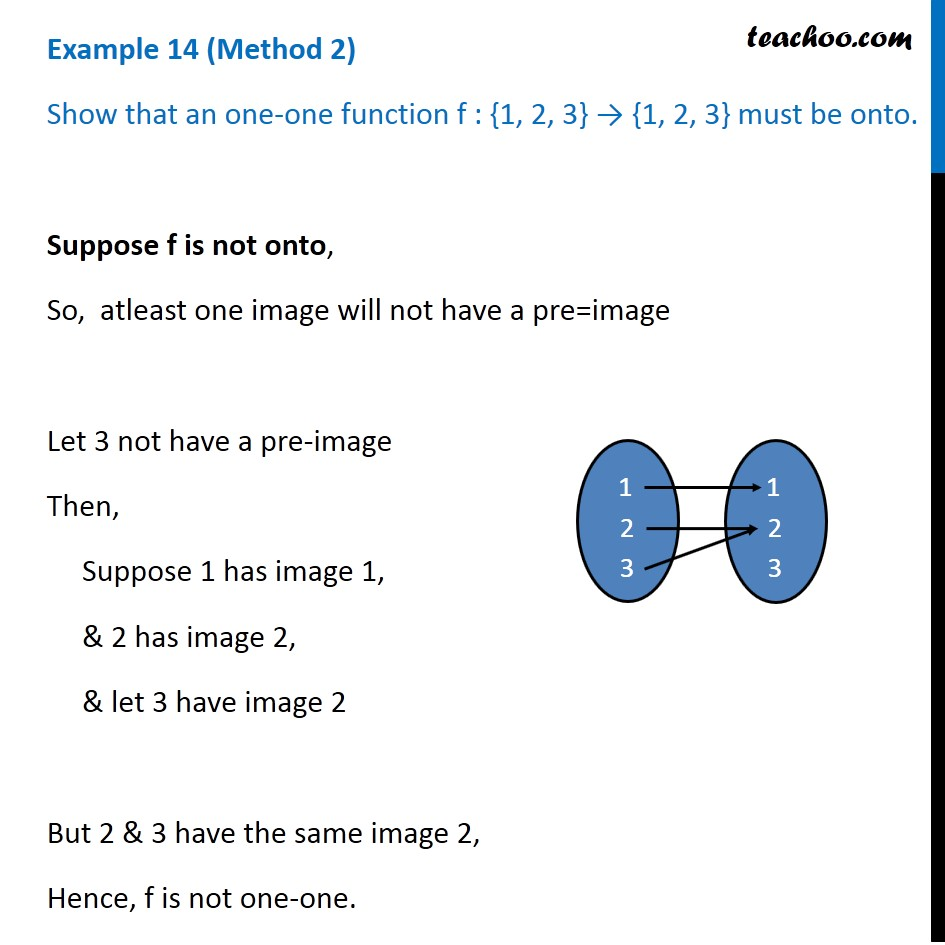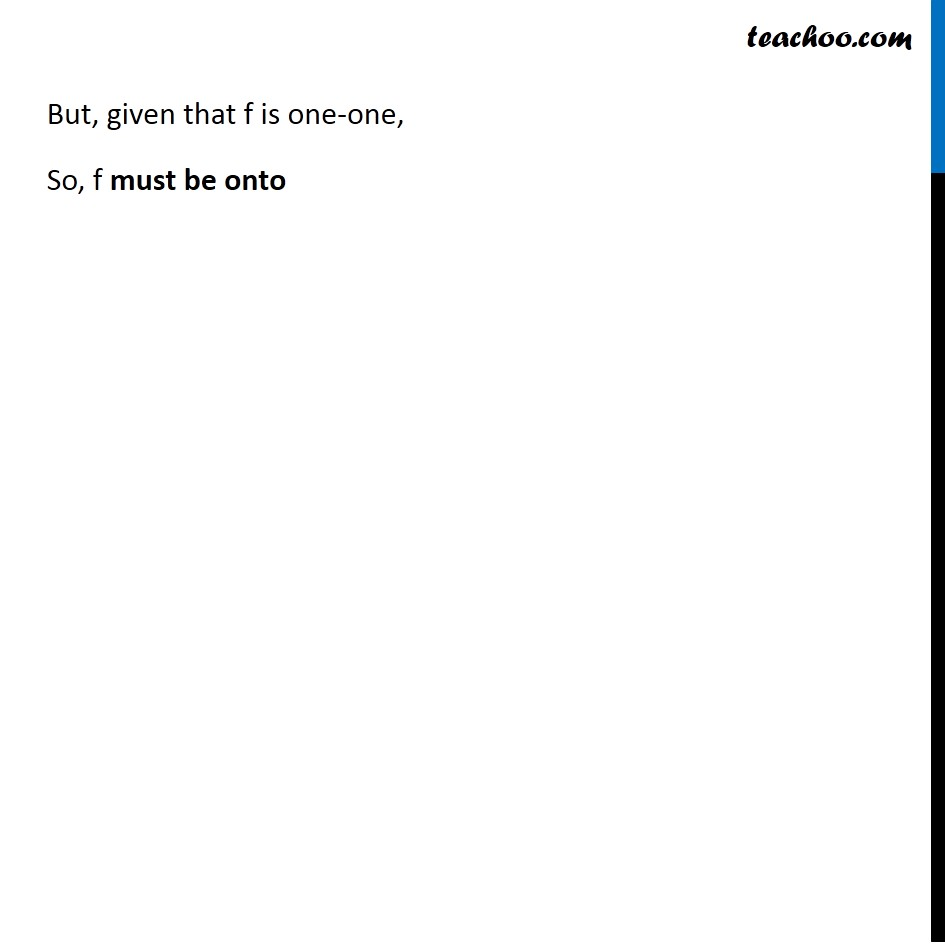1. Chapter 1 Class 12 Relation and Functions (Term 1)
2. Serial order wise
3. Examples

Transcript

Example 14 (Method 1) Show that an one-one function f : {1, 2, 3} → {1, 2, 3} must be onto. Since f is one-one Hence every element 1,2,3 has either of image 1,2,3 and that image is unique Note that in each case, every image has a corresponding element Hence, one-one function f : {1, 2, 3} → {1, 2, 3} is onto. Example 14 (Method 2) Show that an one-one function f : {1, 2, 3} → {1, 2, 3} must be onto. Suppose f is not onto, So, atleast one image will not have a pre=image Let 3 not have a pre-image Then, Suppose 1 has image 1, & 2 has image 2, & let 3 have image 2 But 2 & 3 have the same image 2, Hence, f is not one-one. But, given that f is one-one, So, f must be onto